Next: Implementation Up: Rouche theorem Previous: Rouche theorem   Contents

Mathematical background

Let a system ofequations inunknowns: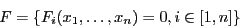We will denote by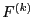the matrix of the derivatives of order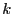of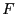with respect to the variable. Let us consider a point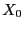and define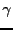as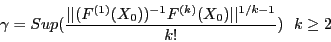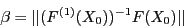and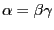. If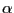is strictly lower than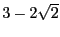, thenhas a single root in a ball centered atwith radius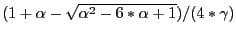and the Newton scheme with initial guesswill converge to the solution.

The most difficult part for using this theorem is to determine. For algebraic equations it is easy to determine a value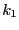, that we will call the order of Rouche theorem, such that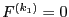and consequentlymay be obtained by computing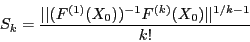for allin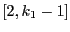and takingas the Sup of all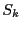.

For non algebraic findingrequires an analysis of the system.

Rouche theorem may be more efficient than Moore or Kantorovitvh theorems. For example when combined with a polynomial deflation (see section 5.9.6) it allows one to solve Wilkinson polynomial of order up to 18 with the C++ arithmetic on a PC, while stand solving procedure fails for order 13.Next: Implementation Up: Rouche theorem Previous: Rouche theorem   Contents
Jean-Pierre Merlet 2012-12-20